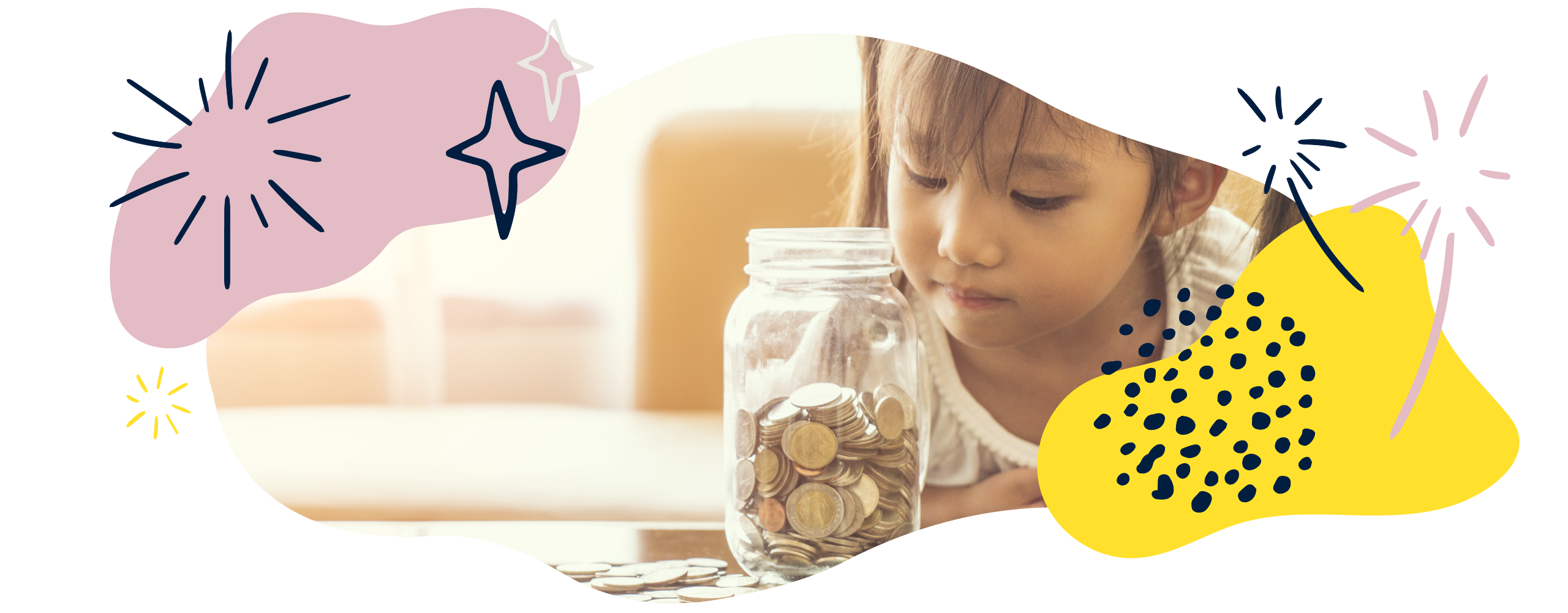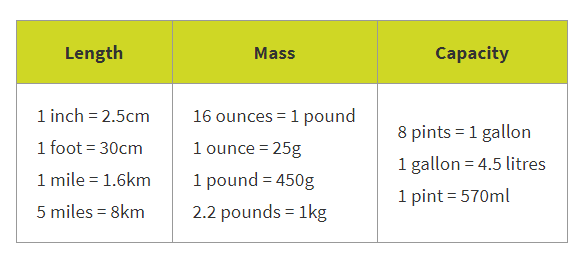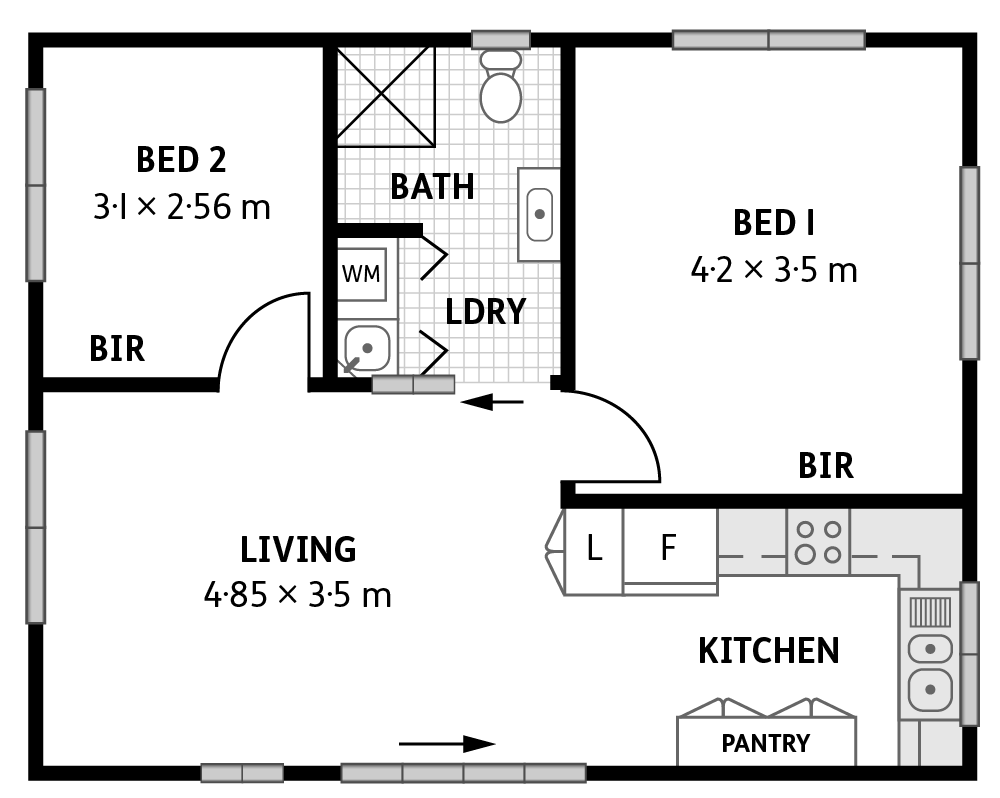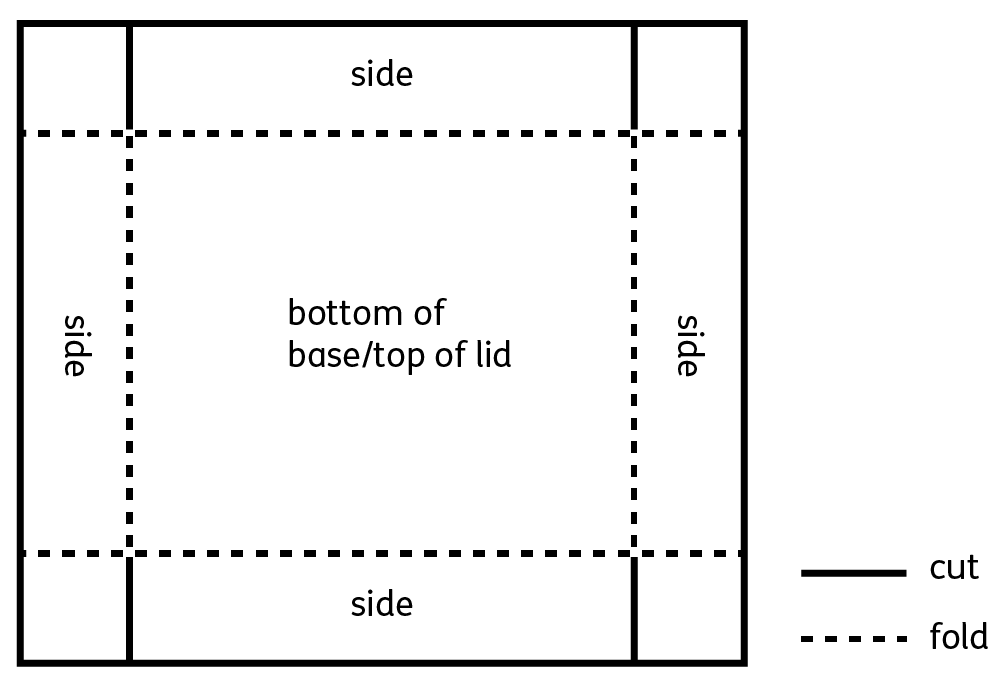We use cookies to enhance your experience on our website. By continuing to use our website, you are agreeing to our use of cookies. You can change your cookie settings at any time. Find out more# Measurement in Year 6 (Ages 10–11)

In Year 6, your child will solve measurement problems using up to three decimal places. They will use simple formulae to calculate area and volume, and will convert between many different units.

The key words for this section are capacityrectilinear, standard unit, and volume.

## What your child will learn

Take a look at the National Curriculum expectations for measurement in Year 6 (age 10–11):

#### Use, read, write, and convert between standard units of measurement

Your child will use, read, write, and convert between standard units of measurement for:

• length
• mass
• volume
• time.

They will be able to convert smaller units of measure into larger units and vice versa. For example, they will be able to convert 1570g to 1.57kg.

Your child will know approximate conversions between metric and imperial units. For example, they will know that there are approximately 25 grams in an ounce and 16 ounces in a pound.

#### Solve problems involving units of measure, using up to 3 decimal places

Your child will solve measurement problems using addition, subtraction, multiplication, and division.

They will solve problems that need them to convert units. For example:

2.3m + 110cm = 340cm

2.3m + 110cm = 3.4m

Your child will use decimal notation up to three decimal places, where appropriate. They should be able to tell if an answer is sensible by estimating their answer before working out the problem precisely.

#### Convert between miles and kilometres

Your child will know that there are approximately 1.6 kilometres in a mile. They will use this to convert between kilometres and miles.

#### Recognise that shapes with the same areas can have different perimeters and vice versa

Your child will recognise that shapes with the same size areas can have different perimeters, and that shapes with the same length perimeters can have different areas.

#### Recognise when to use formulae for the area and volume of shapes

Your child will use formulae to find the area of rectilinear shapes by multiplying length by height.

#### Calculate the area of parallelograms and triangles

Your child will use what they know about finding the area of a rectangle to calculate the area of parallelograms and triangles.

They will do this by splitting big shapes into different smaller shapes. For example, they could find the area of a triangle by finding the area of a square with the same length and height and dividing it by two.

#### Calculate, estimate, and compare the volume of cubes and cuboids using standard units

Your child will calculate, estimate, and compare the volume of cubes and cuboids.

They will record the volume of cubes and cuboids using standard units such as cubic centimetres (cm³), cubic metres (m³), cubic millimetres (mm³), and cubic kilometres (km³).

## How to help at home

There are lots of everyday ways you can help your child to understand measurement. Here are just a few ideas.

### 1. Practise converting between units of measurement

As well as metric units such as metres, grams, and litres, your child will have to use common imperial units such as inches, pounds, and pints. The most common metric units your child will need to convert between are:

• kilometres and metres
• centimetres and metres
• centimetres and millimetres
• grams and kilograms
• litres and millilitres.

You can explore the approximate equivalences between metric and imperial units using a table like this:You can help your child practise conversions by making the most of real-life opportunities. For example, if you are travelling abroad and the distances are measured in kilometres, you could convert these to miles.

Closer to home, think about how many miles it is to the shops and try to convert that into metres, or vice versa.

### 2. Bake!

If you are cooking with your child, discuss which ingredients are measured in metric units and which are measured in imperial units. Can they convert between the two? For example:

The recipe requires half a pound of butter. Can you convert this into grams?

If 1 pound is equivalent to 450g, then half a pound will be equivalent to 225g.Convert units and make a tasty treat!

### 3. Explore area and perimeter

Your child will learn to measure and calculate the perimeter and area of rectangles and composite rectilinear shapesComposite shapes are shapes that can be divided into more than one simple shape. Rectilinear shapes are shapes which only have straight sides and right angles.

Floor plans are great for showing how important measurement is in real-life situations. You could find floor plans for houses or flats online, and ask your child if they can work out the perimeter and area of particular rooms. For example, why not ask your child to calculate the quantity of carpet needed to cover the floor in each bedroom?Once they are comfortable with the idea of a floor plan, you could encourage them to make a floor plan of their dream bedroom! Remind them to think carefully about the room’s scale, and to use squared paper if needed.

They should record their perimeters and areas using the most appropriate units, such as metres and square metres (m²).

### 4. Explore volume

The difference between volume and capacity is quite subtle, and can be difficult to understand:

• Volume means the amount of space occupied by a3Dshape. It is measured in cubed units like cm³.
• Capacitymeans the amount a container can hold. Capacity is measured in metric units such as litres or imperial units such as pints.

You can help your child get to grips with the different units used to measure volume by pointing them out in the real world. When you are at the swimming pool, ask your child whether its capacity is most likely to be measured in cubic millimetres, centimetres, metres, or kilometres, and discuss why.

Another fun activity you can use to explore volume is making boxes. Drawing a plan like the one below onto squared paper, your child could make a number of lidless boxes with different volumes:Find out what happens to the base of each box when you use a smaller piece of paper, and measure the height of the sides of each of the boxes. Can your child work out how much each box can hold? Encourage your child to record and talk about their findings.